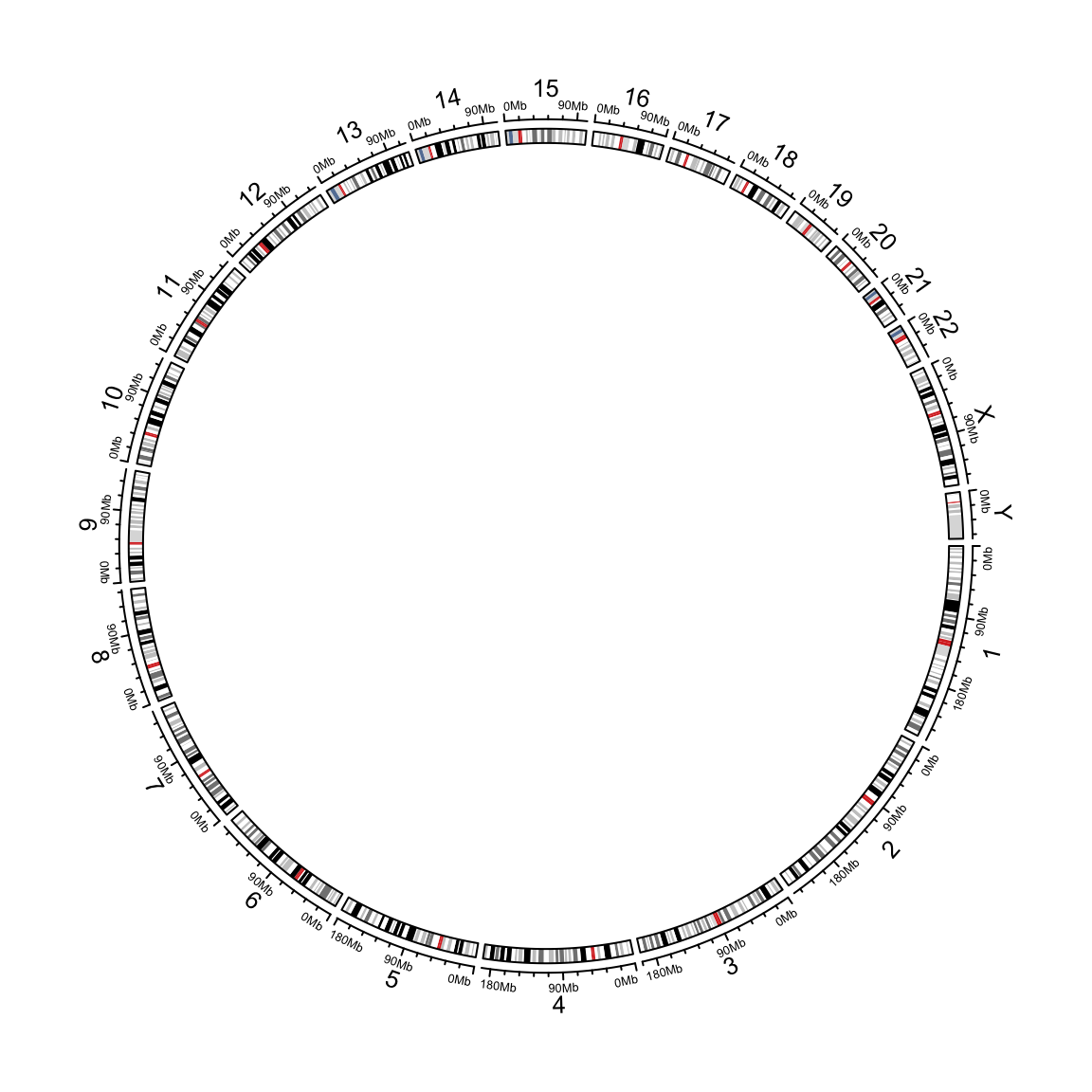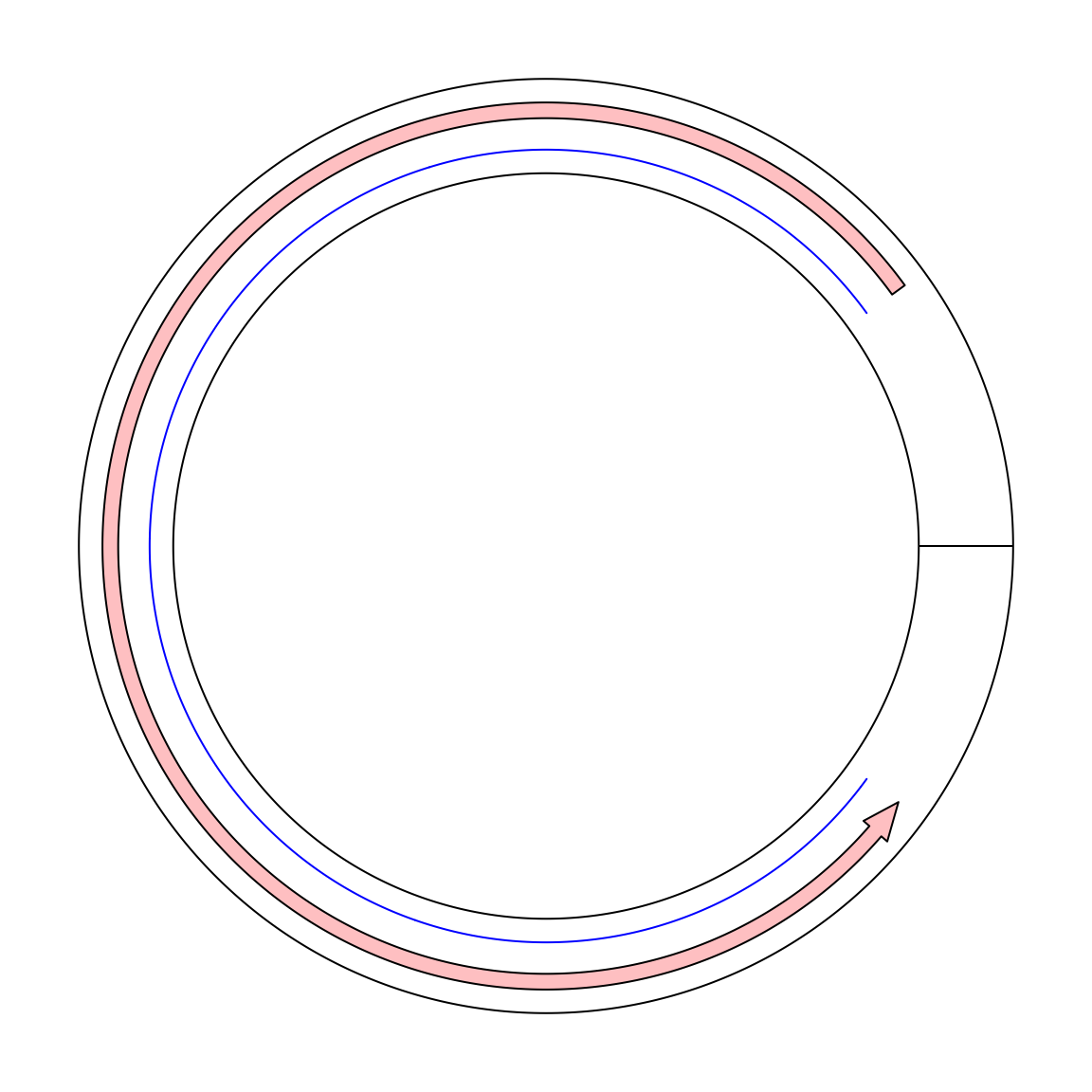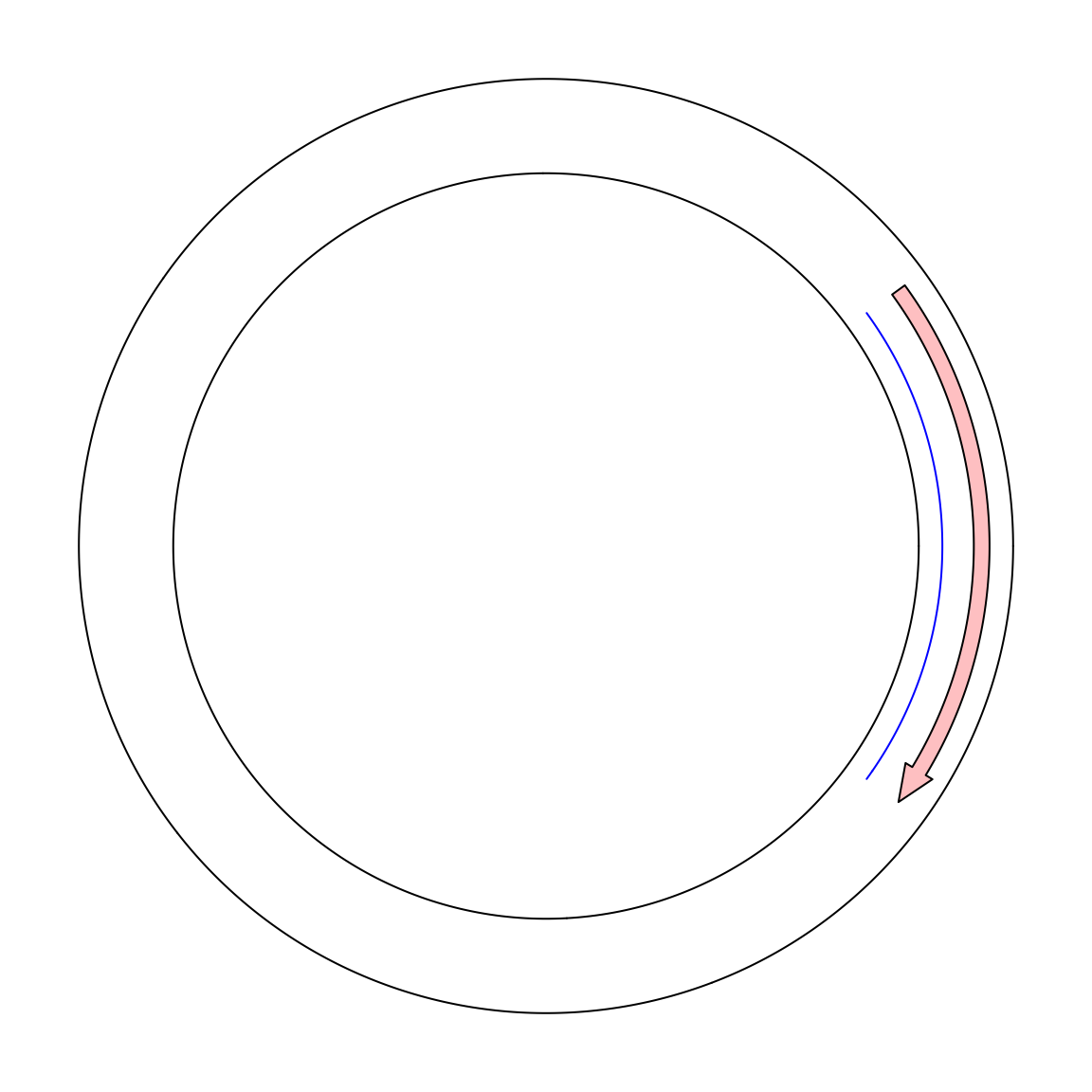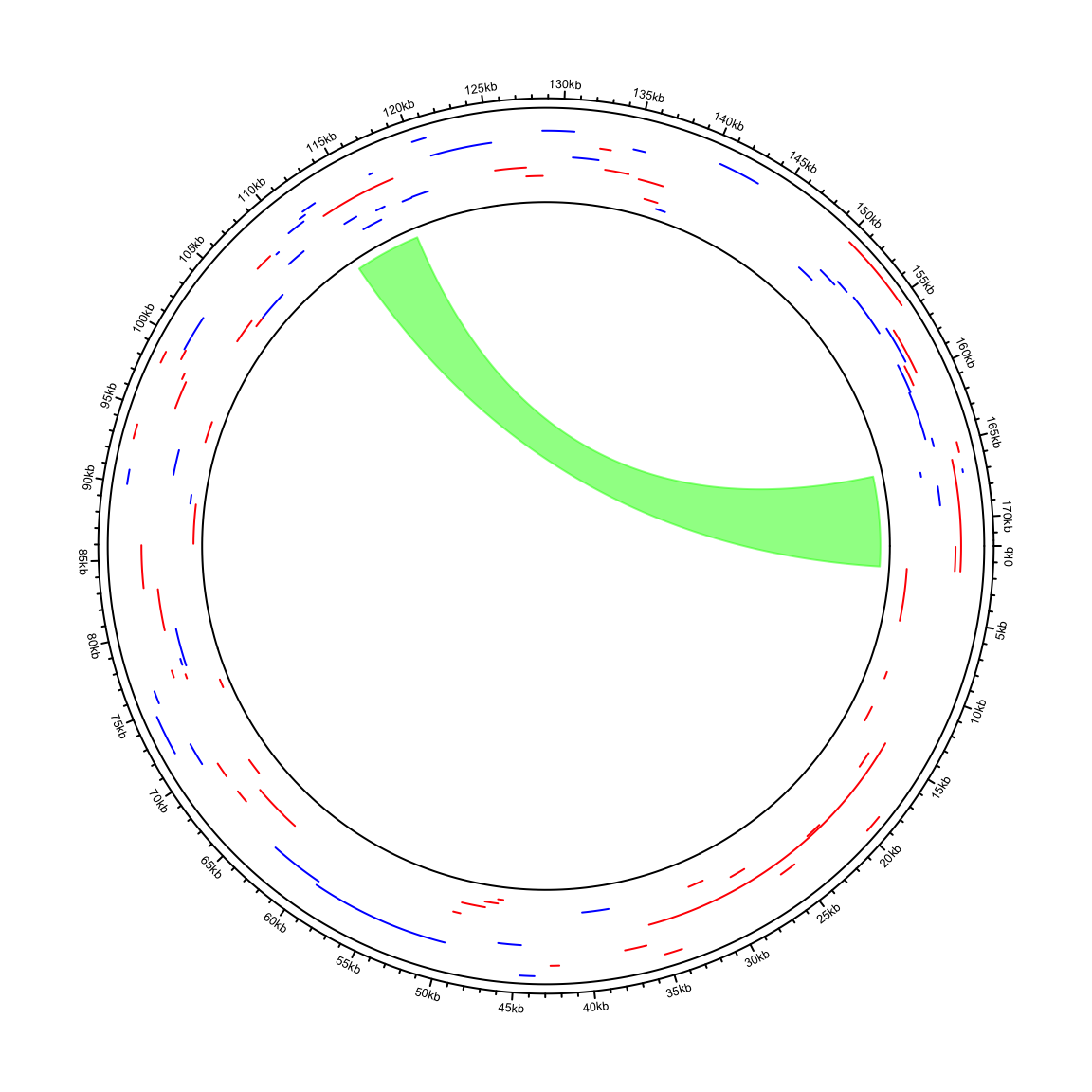# Visualize the 'real' circular genome

circlize applies circular visualization. Essentially, it visualizes data in categories. E.g. for genomic data, the circle is segmented and each segment (or called sector) corresponds to one single chromosome. The following code visualizes 24 chromosomes of human genome.

``````library(circlize)
circos.initializeWithIdeogram()``````Now the question is how to visualize a “real” circular genome where the complete circle corresponds to the genome and the “end” of the genome goes smoothly over the “start” of the genome.

Maybe we can create a single sector and set `gap.degree` to 0. In the following, I tried to draw a line from `x = 9` to `x = 1`. In the circular genome, we expect the line goes through `x = 0`.

``````circos.par(gap.degree = 0, cell.padding = c(0.02, 0, 0.02, 0))
circos.initialize("foo", xlim = c(0, 10))
circos.track(ylim = c(0, 1))
circos.lines(c(9, 1), c(0.2, 0.2), col = "blue")
circos.arrow(9, 1, y = 0.7, width = 0.2)````````circos.clear()``

Unfortunately it is not what we expected. If a line is drawn from `x = 9` to `x = 1` in the normal mode, it only goes reverse close wisely on the circle. And there is also a radical line in the ring which is actually the border of `x = 0` and `x = 10`. Thus in the coordination system, `x = 1` and `x = 9` are still far from each other.

To support the “real” visualization on circular genomes, in circlize, now I add a new mode called “the ring mode”. Simply add `ring = TRUE` in `circos.initialize()`.

``````circos.initialize("foo", xlim = c(0, 10), ring = TRUE)
circos.track(ylim = c(0, 1))
circos.lines(c(9, 1), c(0.2, 0.2), col = "blue")
circos.arrow(9, 1, y = 0.7, width = 0.2)````````circos.clear()``

In the next example, I visualize genome of the Human gammaherpesvirus 4. The genomic coordinates of genes are from https://www.ncbi.nlm.nih.gov/genome/browse/#!/proteins/10261/459250%7CHuman%20gammaherpesvirus%204/. As you can see here, start position in the first row in larger than the end position.

``````df = read.csv("~/Downloads/proteins_10261_459250.csv")
``````##          X.Name   Accession  Start  Stop Strand  GeneID   Locus    Locus.tag
## 1 viral segment NC_007605.1 166103  1680      + 3783751  LMP-2A  HHV4_LMP-2A
## 2 viral segment NC_007605.1     59  1680      + 3783760  LMP-2B  HHV4_LMP-2B
## 3 viral segment NC_007605.1   1736  5692      + 3783722   BNRF1   HHV4_BNRF1
## 4 viral segment NC_007605.1   9675 10187      + 3783689   BCRF1 HHV4_BCRF1.1
## 5 viral segment NC_007605.1  12541 13692      + 3783691   BWRF1 HHV4_BWRF1.1
## 6 viral segment NC_007605.1  14409 35694      + 3783746 EBNA-LP HHV4_EBNA-LP
##   Protein.product Length            Protein.Name
## 1     YP_401631.1    497 membrane protein LMP-2A
## 2     YP_401632.1    378 membrane protein LMP-2B
## 3     YP_401633.1   1318    tegument protein G75
## 4     YP_401634.1    170    interleukin-10 BCRF1
## 5     YP_401635.1    383  protein BWRF1, partial
## 6     YP_401636.1    506 nuclear antigen EBNA-LP``````
``df = df[, 2:5]``

I wrote a helper function `circos.initializeCircularGenome()` especially for circular genomes. In other parts of the code, just use circlize in the normal way.

``````circos.initializeCircularGenome("NC_007605.1", genome_size = 171823)
circos.genomicTrack(df, ylim = c(0, 1), panel.fun = function(region, value, ...) {
circos.genomicLines(region, value = runif(nrow(region)), type = "segment",
col = ifelse(value[, 1] == "+", "Red", "blue"))
})
circos.genomicLink(df[1, ], df[60, ], col = "#00FF0080")``````